# NCERT Exemplar Class 12 Physics Solutions for Chapter 8 Electromagnetic Waves

The NCERT Class 12 Physics Exemplar for Chapter 8 Electromagnetic Waves is prepared by subject experts of BYJU’S by using the NCERT Class 12 Exemplar Physics book, sample papers, previous years’ questions, worksheets and Class 12 Physics NCERT examples.

The NCERT Class 12 Physics Exemplar for Chapter 8 PDF has MCQs, fill in the blanks, numerical problems, worksheets, HOTS, and tips and tricks that will help you understand the topics and score good marks in the CBSE Board Class 12 examination and entrance exams, like NEET and JEE.

## Download the PDF of NCERT Exemplar for Class 12 Physics Chapter 8 Electromagnetic Waves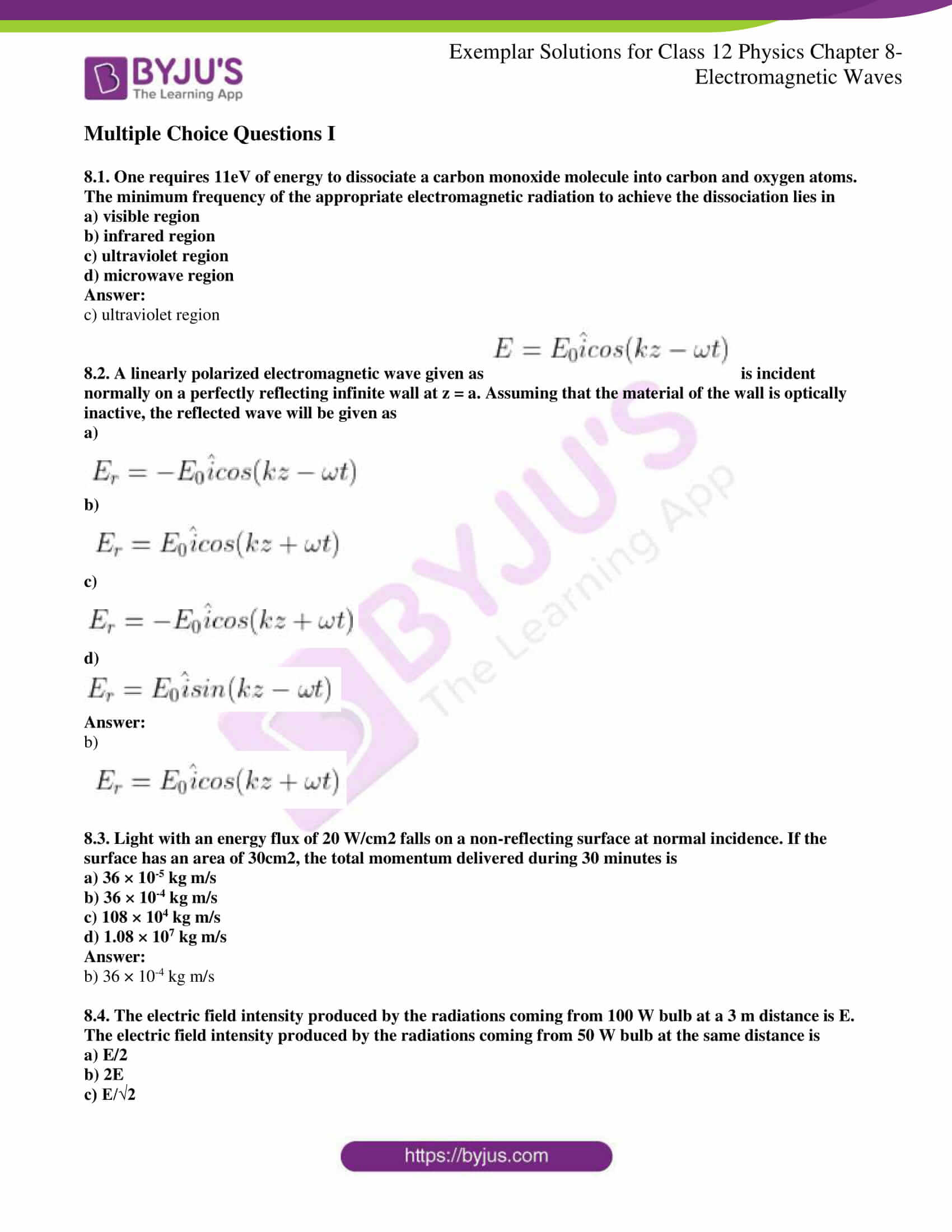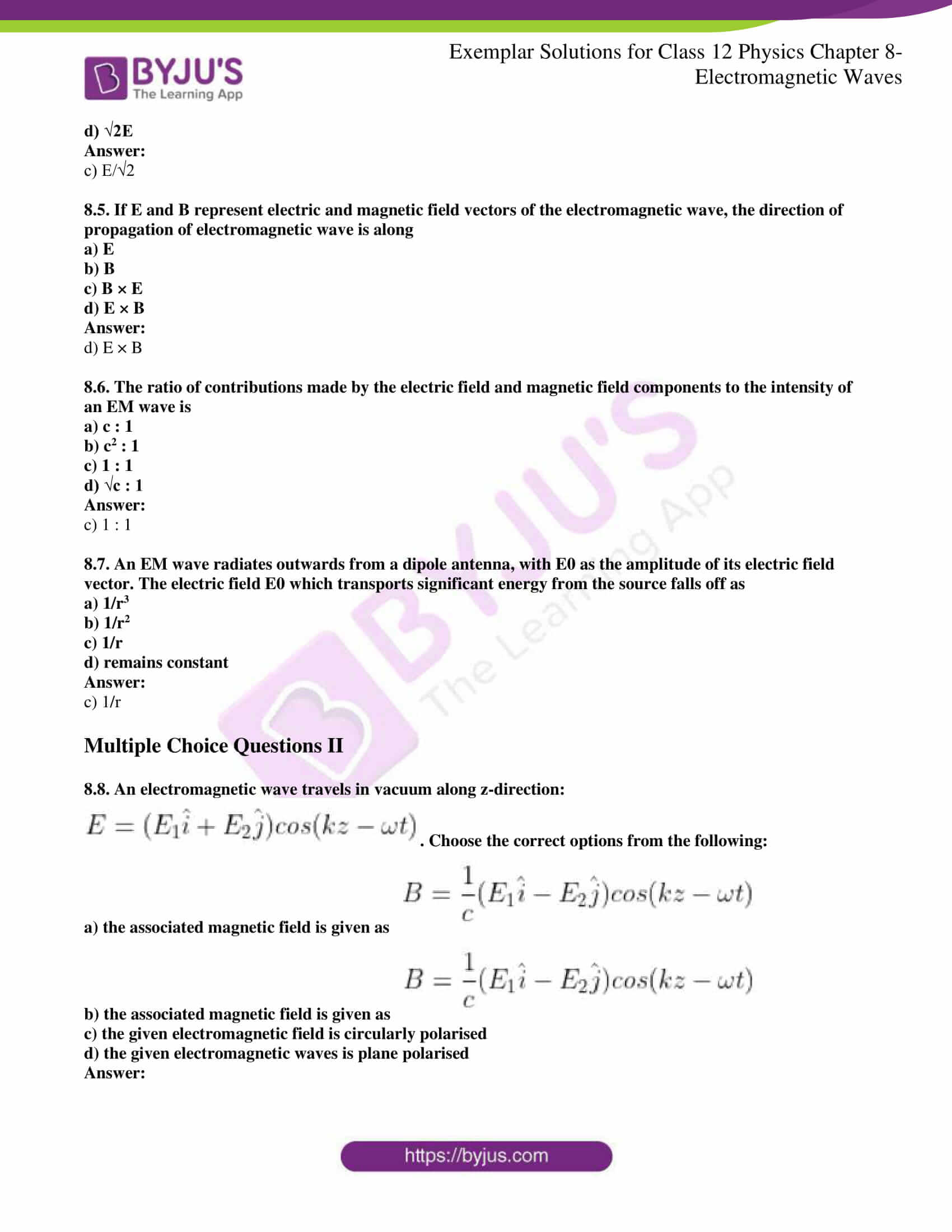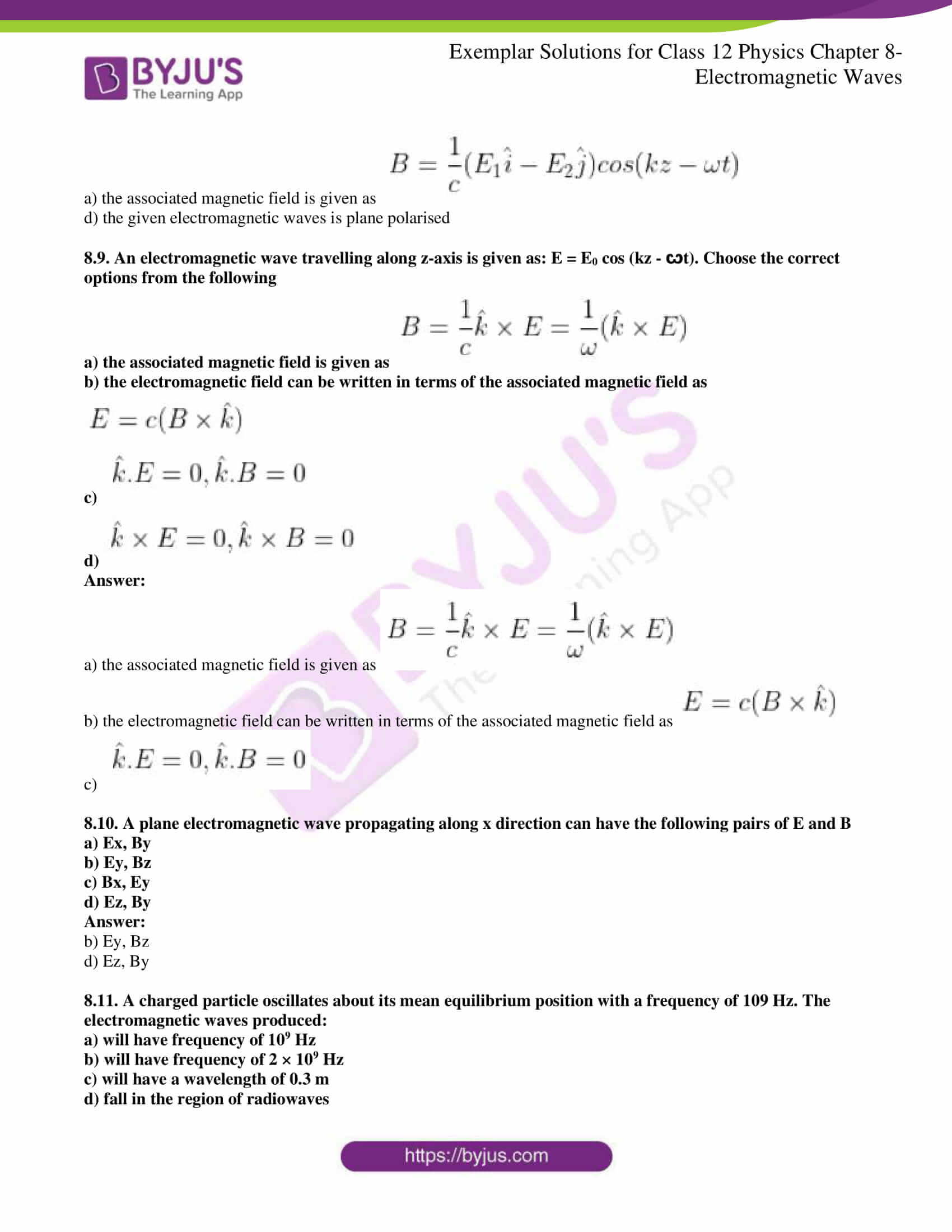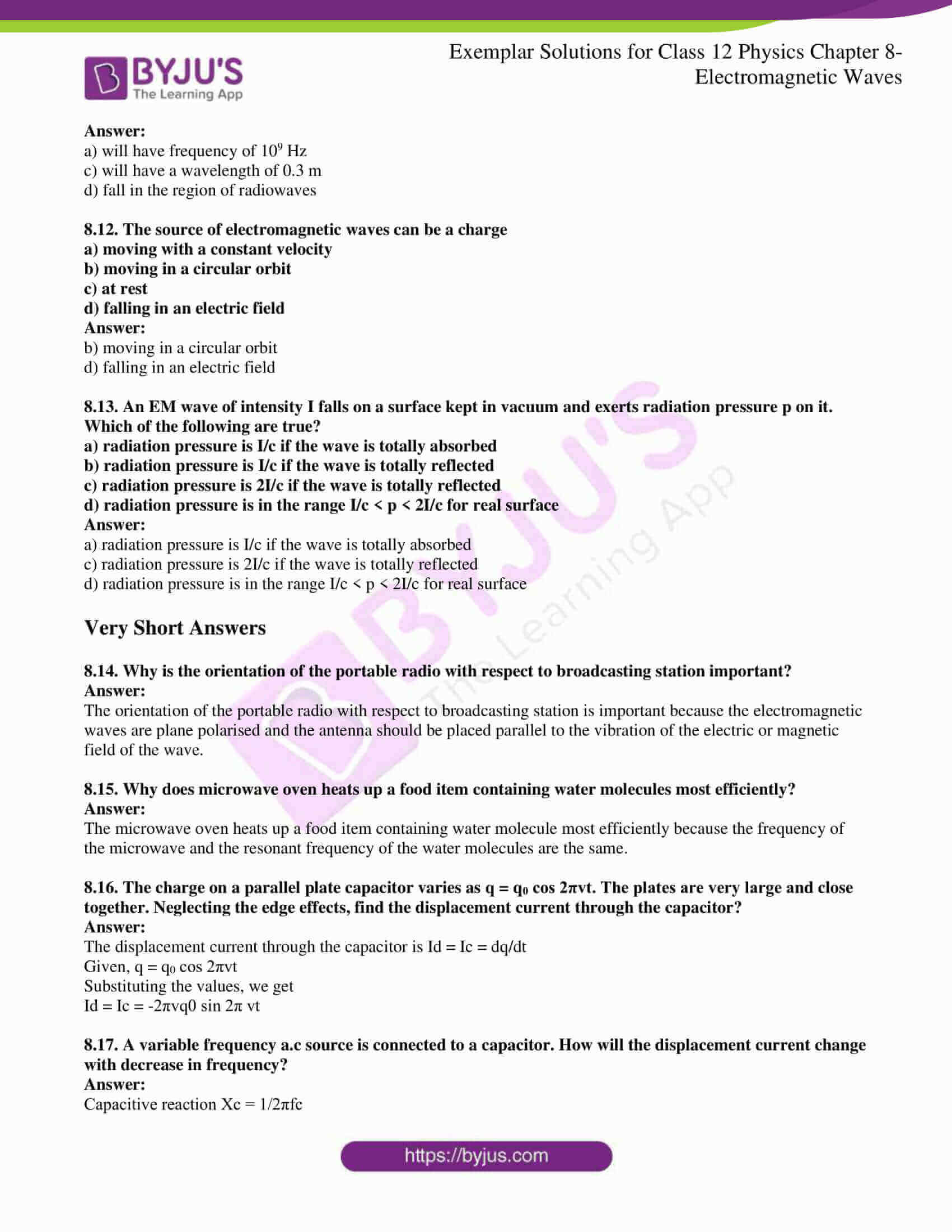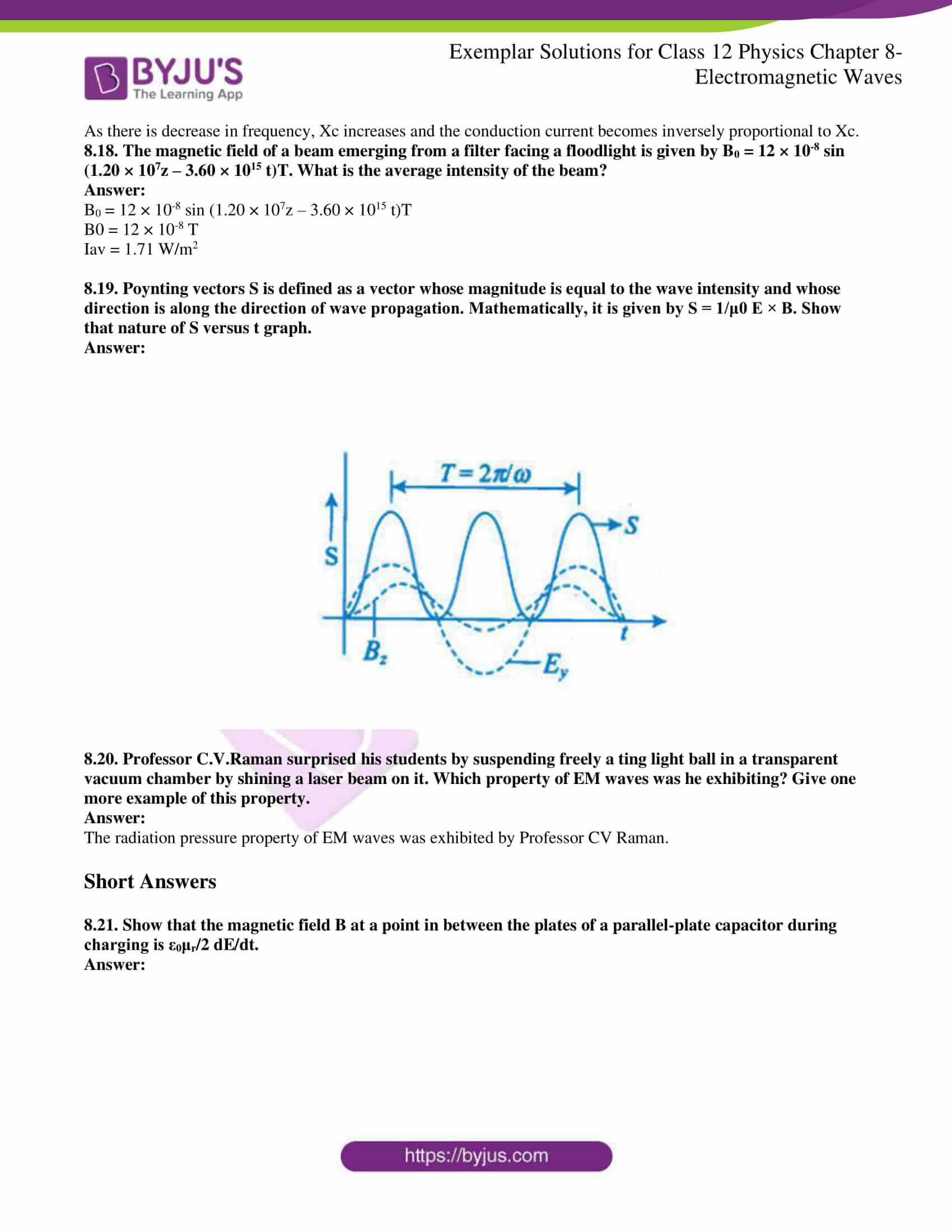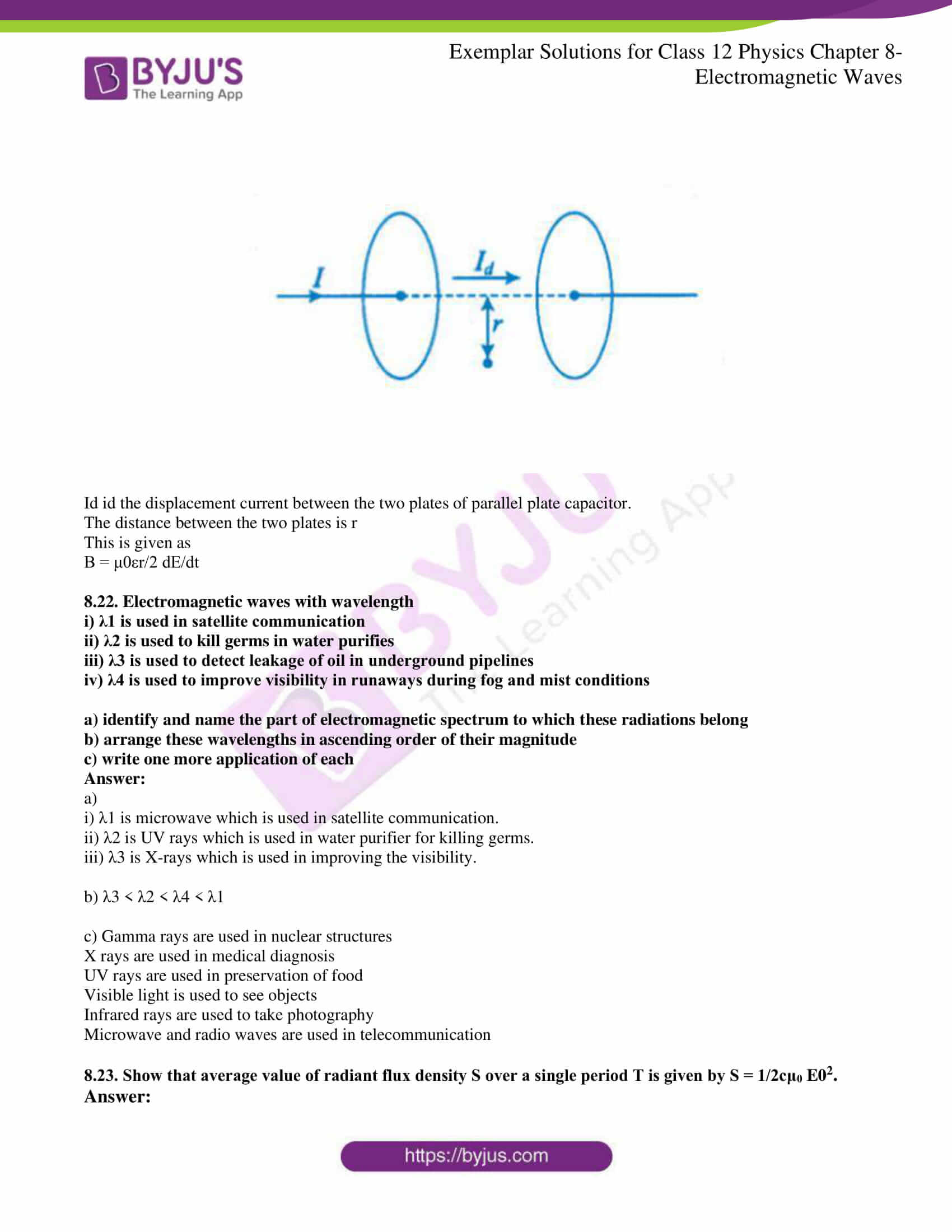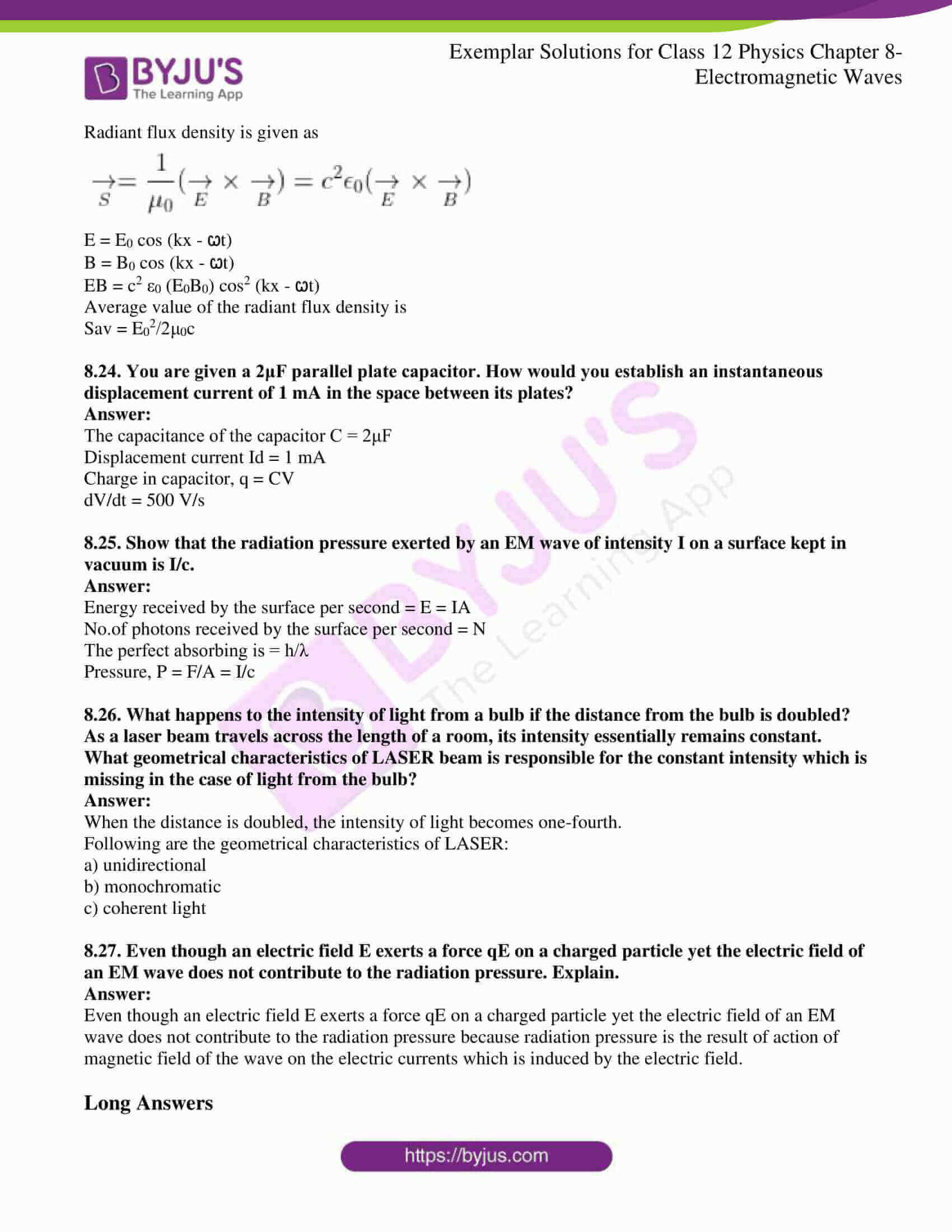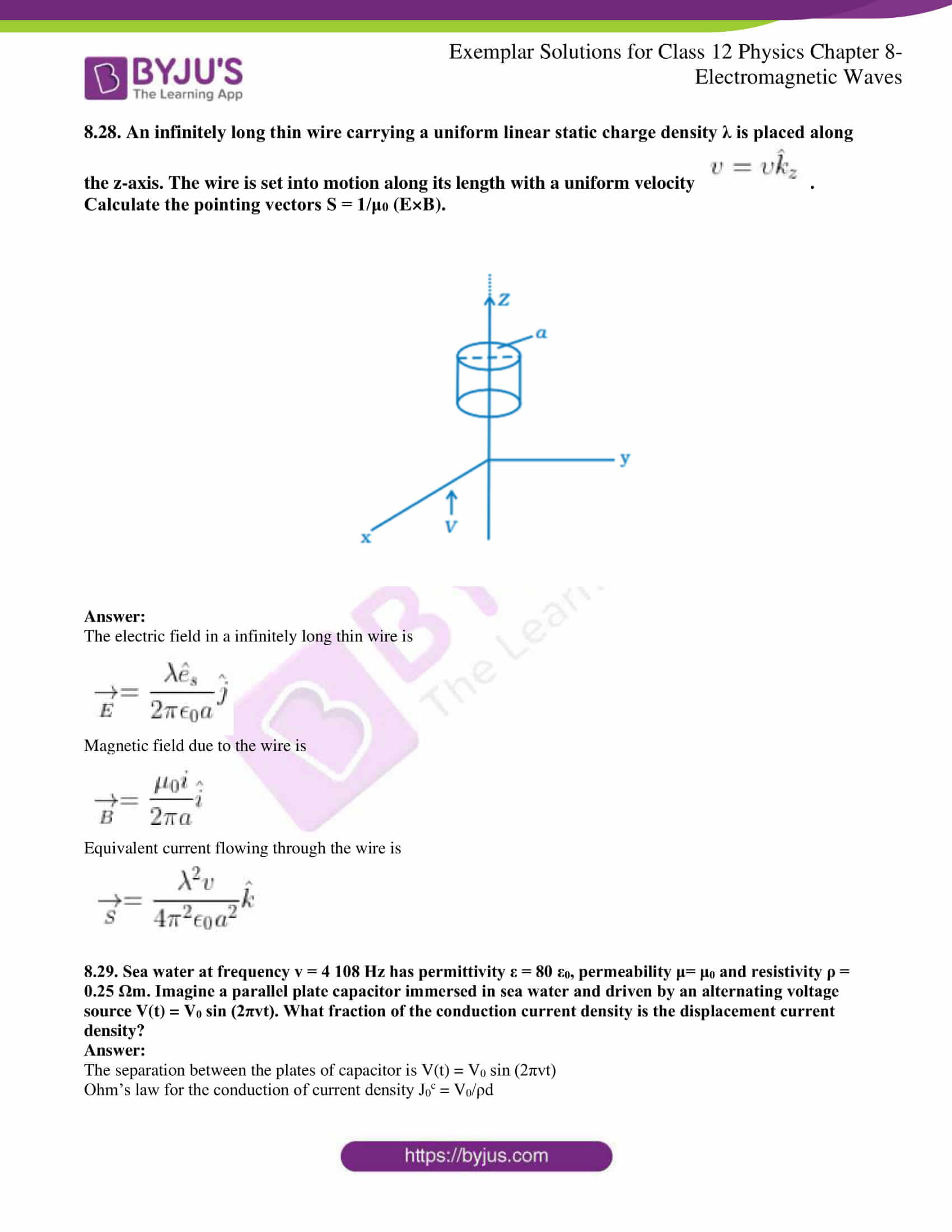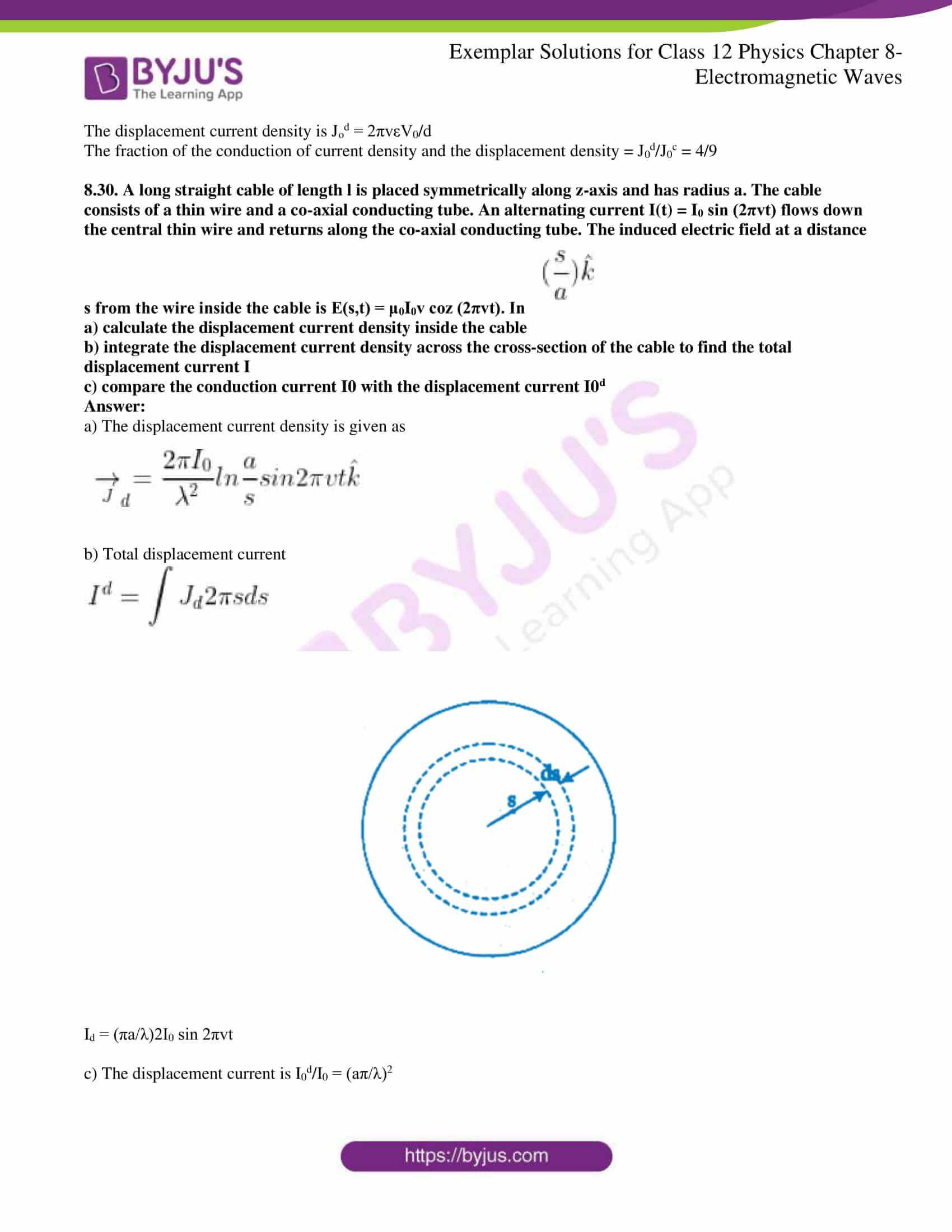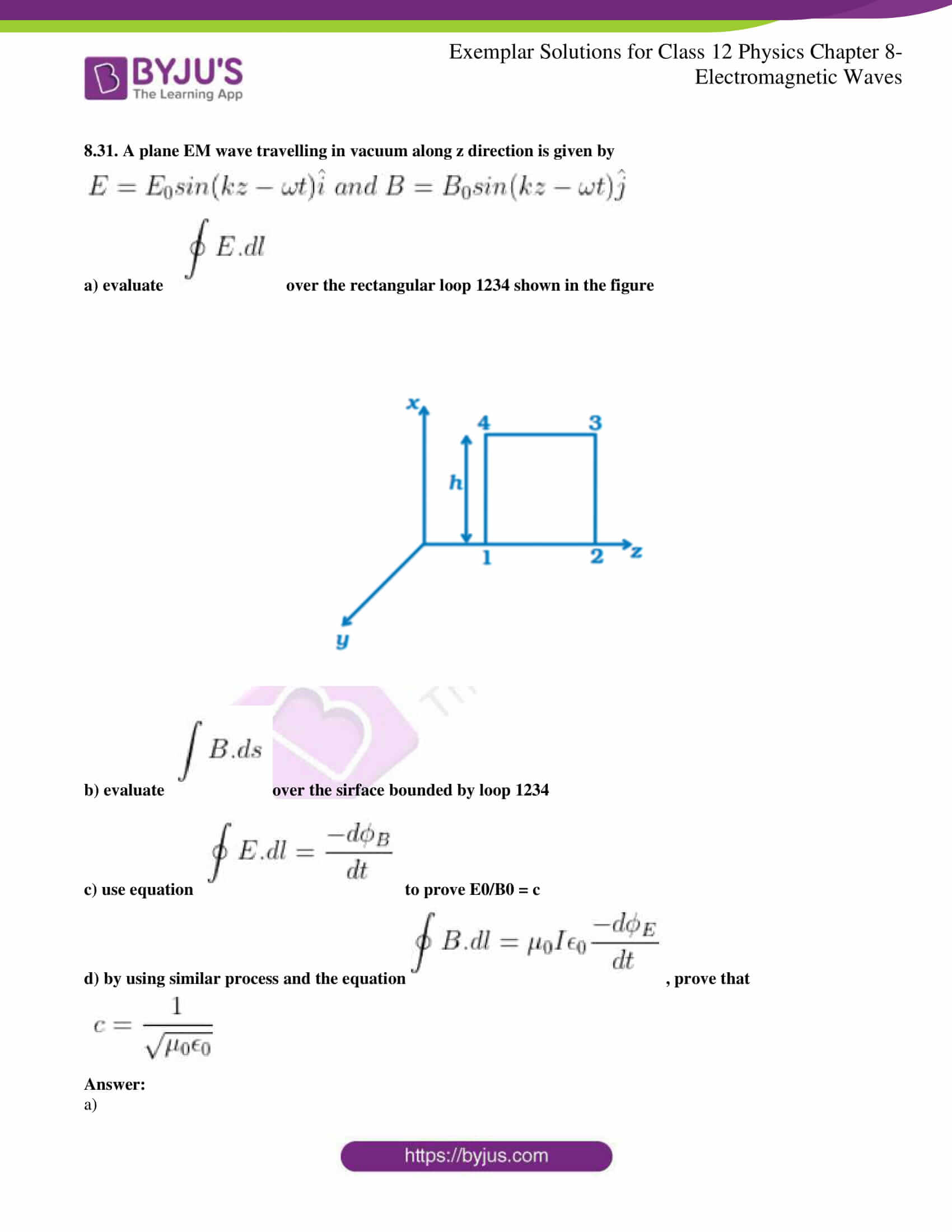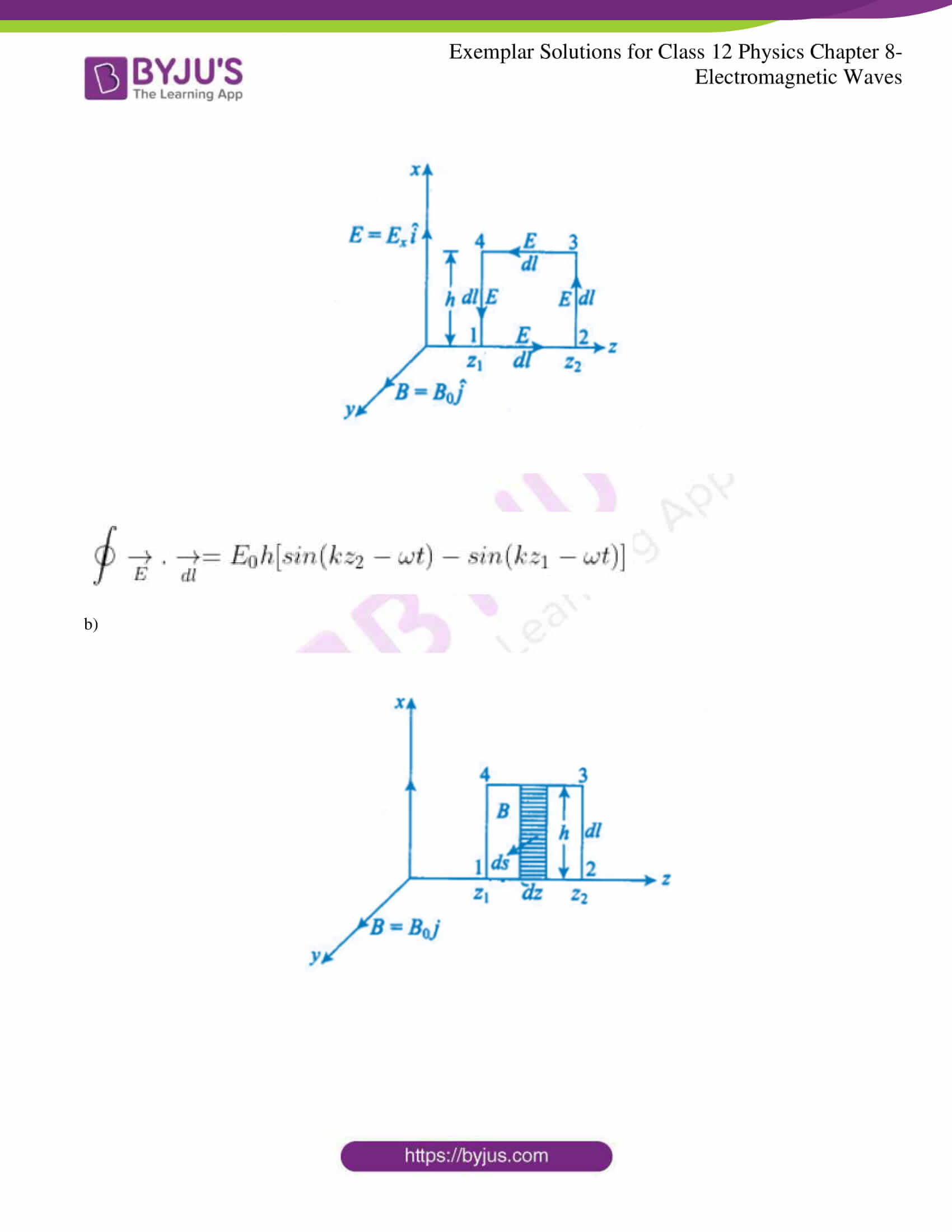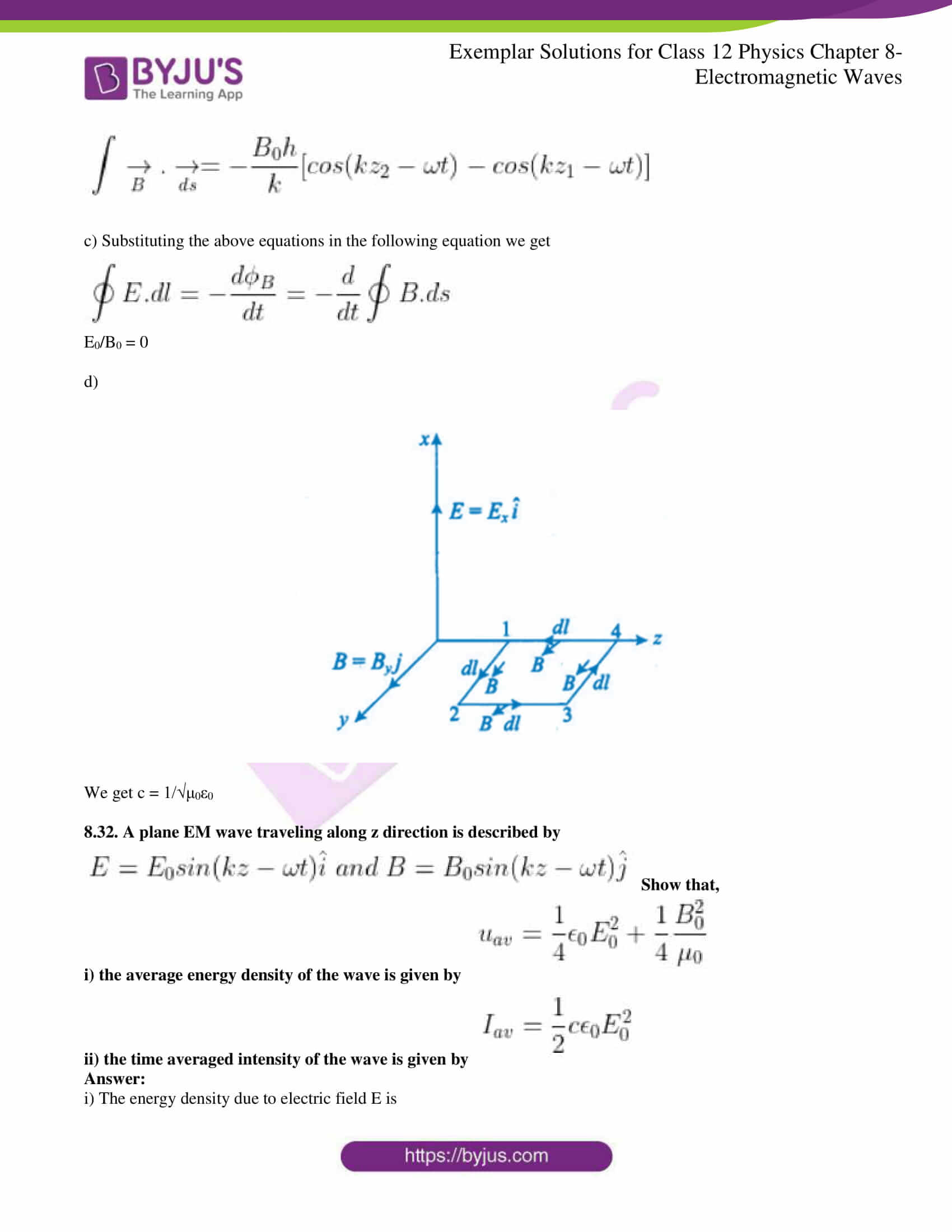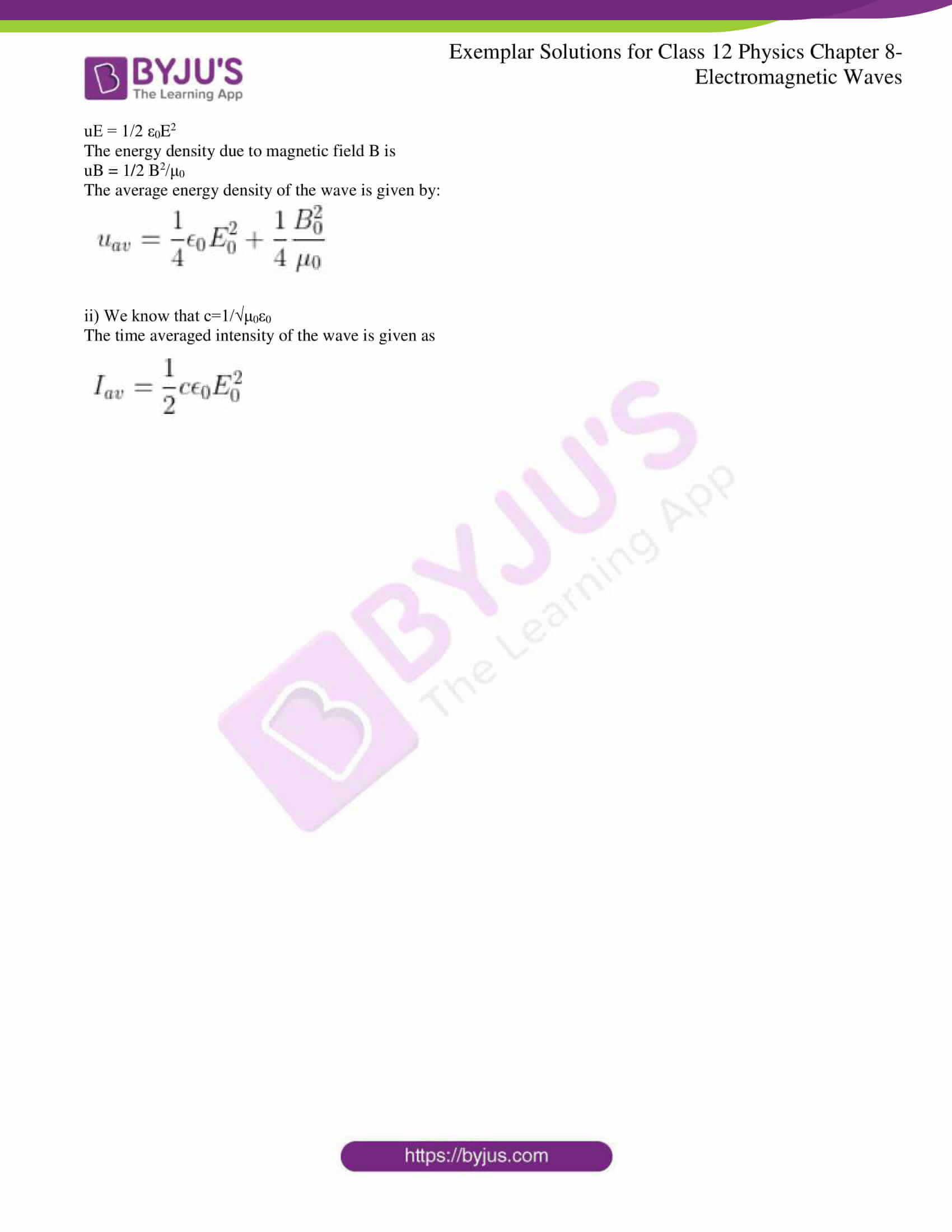### Multiple Choice Questions I

8.1. One requires 11eV of energy to dissociate a carbon monoxide molecule into carbon and oxygen atoms. The minimum frequency of the appropriate electromagnetic radiation to achieve the dissociation lies in

a) visible region

b) infrared region

c) ultraviolet region

d) microwave region

c) ultraviolet region

8.2. A linearly polarized electromagnetic wave given as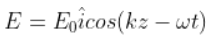is incident normally on a perfectly reflecting infinite wall at z = a. Assuming that the material of the wall is optically inactive, the reflected wave will be given as

a)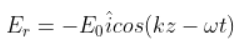b)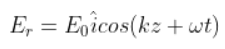c)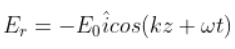d)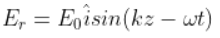b)8.3. Light with an energy flux of 20 W/cm2 falls on a non-reflecting surface at normal incidence. If the surface has an area of 30cm2, the total momentum delivered during 30 minutes is

a) 36 × 10-5 kg m/s

b) 36 × 10-4 kg m/s

c) 108 × 104 kg m/s

d) 1.08 × 107 kg m/s

b) 36 × 10-4 kg m/s

8.4. The electric field intensity produced by the radiations coming from 100 W bulb at a 3 m distance is E. The electric field intensity produced by the radiations coming from 50 W bulb at the same distance is

a) E/2

b) 2E

c) E/√2

d) √2E

c) E/√2

8.5. If E and B represent electric and magnetic field vectors of the electromagnetic wave, the direction of propagation of the electromagnetic wave is along

a) E

b) B

c) B × E

d) E × B

d) E × B

8.6. The ratio of contributions made by the electric field and magnetic field components to the intensity of an EM wave is

a) c: 1

b) c2 : 1

c) 1: 1

d) √c: 1

c) 1: 1

8.7. An EM wave radiates outwards from a dipole antenna, with E0 as the amplitude of its electric field vector. The electric field E0 which transports significant energy from the source falls off as

a) 1/r3

b) 1/r2

c) 1/r

d) remains constant

c) 1/r

### Multiple Choice Questions II

8.8. An electromagnetic wave travels in vacuum along z-direction: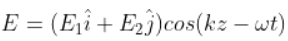. Choose the correct options from the following:

a) the associated magnetic field is given as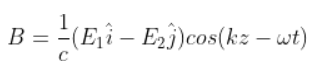b) the associated magnetic field is given asc) the given electromagnetic field is circularly polarised

d) the given electromagnetic waves is plane polarised

a) the associated magnetic field is given asd) the given electromagnetic waves is plane polarised

8.9. An electromagnetic wave travelling along the z-axis is given as: E = E0 cos (kz – ꞷt). Choose the correct options from the following

a) the associated magnetic field is given as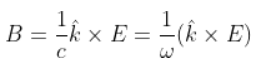b) the electromagnetic field can be written in terms of the associated magnetic field as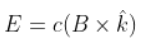c)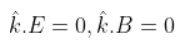d)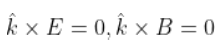a) the associated magnetic field is given asb) the electromagnetic field can be written in terms of the associated magnetic field asc)8.10. A plane electromagnetic wave propagating along x-direction can have the following pairs of E and B

a) Ex, By

b) Ey, Bz

c) Bx, Ey

d) Ez, By

b) Ey, Bz

d) Ez, By

8.11. A charged particle oscillates about its mean equilibrium position with a frequency of 109 Hz. The electromagnetic waves produced:

a) will have a frequency of 109 Hz

b) will have a frequency of 2 × 109 Hz

c) will have a wavelength of 0.3 m

d) fall in the region of radiowaves

a) will have a frequency of 109 Hz

c) will have a wavelength of 0.3 m

d) fall in the region of radiowaves

8.12. The source of electromagnetic waves can be a charge

a) moving with a constant velocity

b) moving in a circular orbit

c) at rest

d) falling in an electric field

b) moving in a circular orbit

d) falling in an electric field

8.13. An EM wave of intensity I falls on a surface kept in vacuum and exerts radiation pressure p on it. Which of the following are true?

a) radiation pressure is I/c if the wave is totally absorbed

b) radiation pressure is I/c if the wave is totally reflected

c) radiation pressure is 2I/c if the wave is totally reflected

d) radiation pressure is in the range I/c < p < 2I/c for real surface

a) radiation pressure is I/c if the wave is totally absorbed

c) radiation pressure is 2I/c if the wave is totally reflected

d) radiation pressure is in the range I/c < p < 2I/c for real surface

8.14. Why is the orientation of the portable radio with respect to broadcasting station important?

The orientation of the portable radio with respect to the broadcasting station is important because the electromagnetic waves are plane polarised and the antenna should be placed parallel to the vibration of the electric or magnetic field of the wave.

8.15. Why does a microwave oven heats up a food item containing water molecules most efficiently?

The microwave oven heats up a food item containing water molecule most efficiently because the frequency of the microwave and the resonant frequency of the water molecules are the same.

8.16. The charge on a parallel plate capacitor varies as q = q0 cos 2πvt. The plates are very large and close together. Neglecting the edge effects, find the displacement current through the capacitor?

The displacement current through the capacitor is Id = Ic = dq/dt

Given, q = q0 cos 2πvt

Substituting the values, we get

Id = Ic = -2πvq0 sin 2π vt

8.17. A variable frequency a.c source is connected to a capacitor. How will the displacement current change with a decrease in frequency?

Capacitive reaction Xc = 1/2πfc

As there is a decrease in frequency, Xc increases and the conduction current becomes inversely proportional to Xc.

8.18. The magnetic field of a beam emerging from a filter facing a floodlight is given by B0 = 12 × 10-8 sin (1.20 × 107z – 3.60 × 1015 t)T. What is the average intensity of the beam?

B0 = 12 × 10-8 sin (1.20 × 107z – 3.60 × 1015 t)T

B0 = 12 × 10-8 T

Iav = 1.71 W/m2

8.19. Poynting vectors S is defined as a vector whose magnitude is equal to the wave intensity and whose direction is along the direction of wave propagation. Mathematically, it is given by S = 1/μ0 E × B. Show that nature of S versus t graph.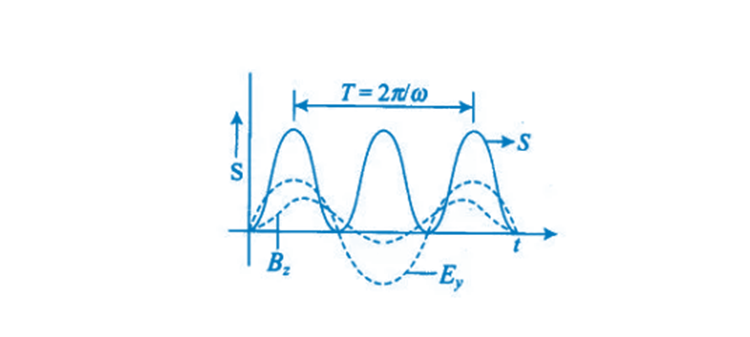8.20. Professor C.V.Raman surprised his students by suspending freely a ting light ball in a transparent vacuum chamber by shining a laser beam on it. Which property of EM waves was he exhibiting? Give one more example of this property.

The radiation pressure property of EM waves was exhibited by Professor CV Raman.

8.21. Show that the magnetic field B at a point in between the plates of a parallel-plate capacitor during charging is ε0μr/2 dE/dt.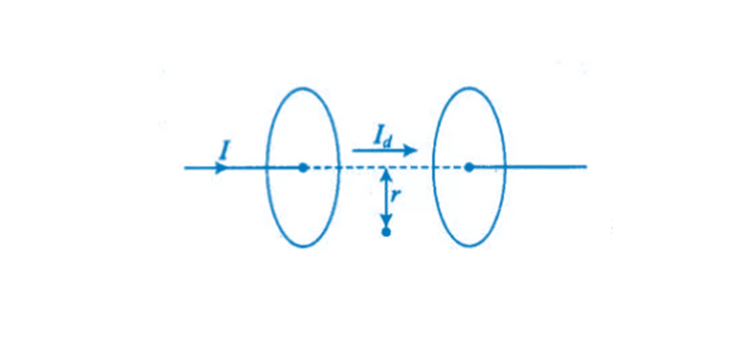Id is the displacement current between the two plates of a parallel plate capacitor.

The distance between the two plates is r

This is given as

B = μ0εr/2 dE/dt

8.22. Electromagnetic waves with wavelength

i) λ1 is used in satellite communication

ii) λ2 is used to kill germs in water purifies

iii) λ3 is used to detect leakage of oil in underground pipelines

iv) λ4 is used to improve visibility in runaways during fog and mist conditions

a) identify and name the part of the electromagnetic spectrum to which these radiations belong

b) arrange these wavelengths in ascending order of their magnitude

c) write one more application of each

a)

i) λ1 is a microwave which is used in satellite communication.

ii) λ2 is UV rays which is used in a water purifier for killing germs.

iii) λ3 is X-rays which is used in improving the visibility.

b) λ3 < λ2 < λ4 < λ1

c) Gamma rays are used in nuclear structures

X rays are used in medical diagnosis

UV rays are used in the preservation of food

Visible light is used to see objects

Infrared rays are used to take photography

Microwave and radio waves are used in telecommunication

8.23. Show that the average value of radiant flux density S over a single period T is given by S = 1/2cμ0 E02.

Radiant flux density is given as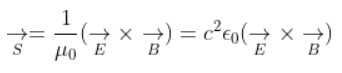E = E0 cos (kx – ꞷt)

B = B0 cos (kx – ꞷt)

EB = c2 ε0 (E0B0) cos2 (kx – ꞷt)

Average value of the radiant flux density is

Sav = E02/2μ0c

8.24. You are given a 2μF parallel plate capacitor. How would you establish an instantaneous displacement current of 1 mA in the space between its plates?

The capacitance of the capacitor C = 2μF

Displacement current Id = 1 mA

Charge in capacitor, q = CV

dV/dt = 500 V/s

8.25. Show that the radiation pressure exerted by an EM wave of intensity I on a surface kept in vacuum is I/c.

Energy received by the surface per second = E = IA

No.of photons received by the surface per second = N

The perfect absorbing is = h/λ

Pressure, P = F/A = I/c

8.26. What happens to the intensity of light from a bulb if the distance from the bulb is doubled? As a laser beam travels across the length of a room, its intensity essentially remains constant. What geometrical characteristics of the LASER beam is responsible for the constant intensity which is missing in the case of light from the bulb?

When the distance is doubled, the intensity of light becomes one-fourth.

Following are the geometrical characteristics of the LASER:

a) unidirectional

b) monochromatic

c) coherent light

8.27. Even though an electric field E exerts a force qE on a charged particle yet the electric field of an EM wave does not contribute to the radiation pressure. Explain.

Even though an electric field E exerts a force qE on a charged particle yet the electric field of an EM wave does not contribute to the radiation pressure because radiation pressure is the result of the action of the magnetic field of the wave on the electric currents which is induced by the electric field.

8.28. An infinitely long thin wire carrying a uniform linear static charge density λ is placed along the z-axis. The wire is set into motion along its length with a uniform velocity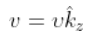. Calculate the pointing vectors S = 1/μ0 (E×B).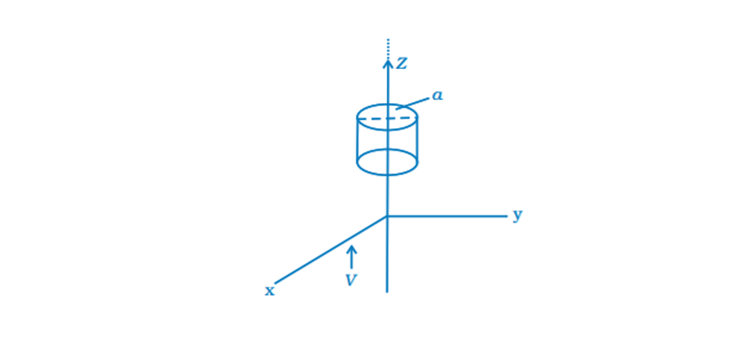The electric field in an infinitely long thin wire is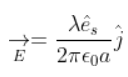Magnetic field due to the wire is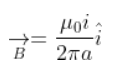The equivalent current flowing through the wire is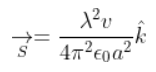8.29. Seawater at frequency v = 4 108 Hz has permittivity ε = 80 ε0, permeability μ= μ0 and resistivity ρ = 0.25 Ωm. Imagine a parallel plate capacitor immersed in seawater and driven by an alternating voltage source V(t) = V0 sin (2πvt). What fraction of the conduction current density is the displacement current density?

The separation between the plates of the capacitor is V(t) = V0 sin (2πvt)

Ohm’s law for the conduction of current density J0c = V0/ρd

The displacement current density is Jod = 2πvεV0/d

The fraction of the conduction of current density and the displacement density = J0d/J0c = 4/9

8.30. A long straight cable of length l is placed symmetrically along the z-axis and has radius a. The cable consists of a thin wire and a co-axial conducting tube. An alternating current I(t) = I0 sin (2πvt) flows down the central thin wire and returns along the co-axial conducting tube. The induced electric field at a distance s from the wire inside the cable is E(s,t) = μ0I0v coz (2πvt). In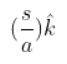a) calculate the displacement current density inside the cable

b) integrate the displacement current density across the cross-section of the cable to find the total displacement current I

c) compare the conduction current I0 with the displacement current I0d

a) The displacement current density is given as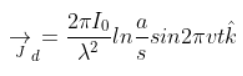b) Total displacement current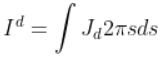Id = (πa/λ)2I0 sin 2πvt

c) The displacement current is I0d/I0 = (aπ/λ)2

8.31. A plane EM wave travelling in vacuum along z-direction is given by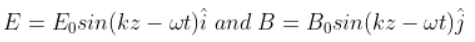a) evaluate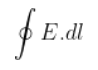over the rectangular loop 1234 shown in the figure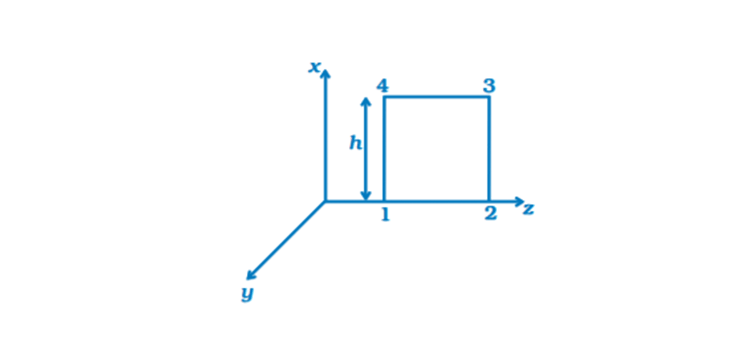b) evaluate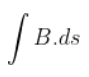over the surface bounded by loop 1234

c) use equation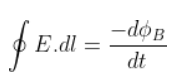to prove E0/B0 = c

d) by using a similar process and the equation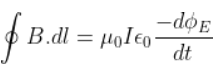, prove that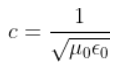a)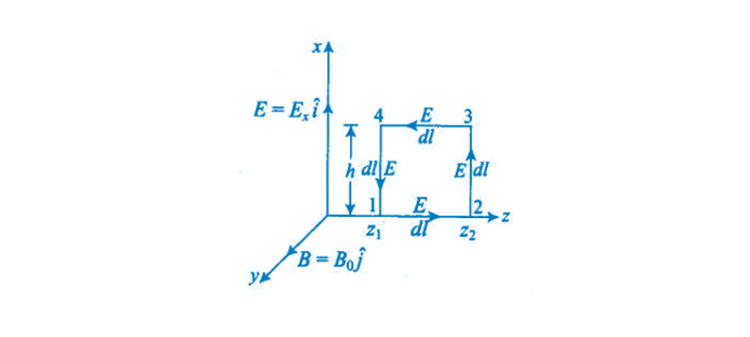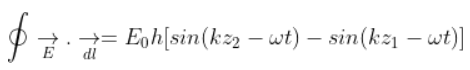b)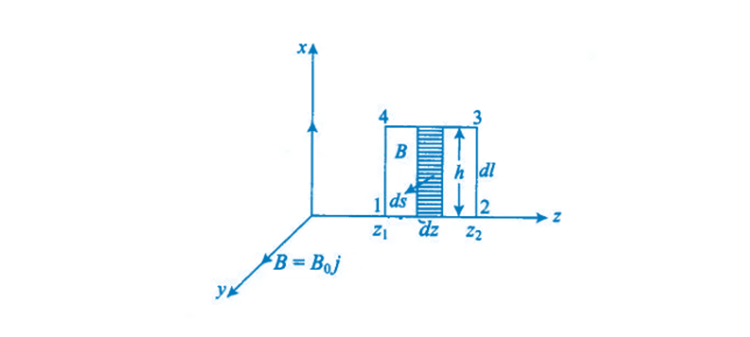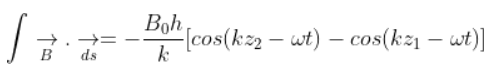c) Substituting the above equations in the following equation we get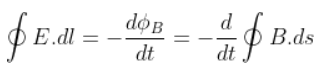E0/B0 = 0

d)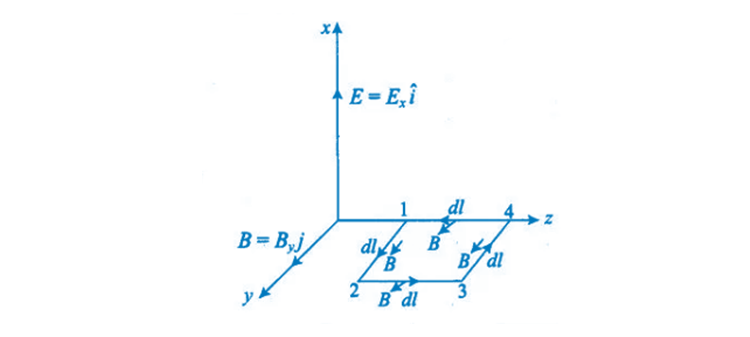We get c = 1/√μ0ε0

8.32. A plane EM wave travelling along z-direction is described by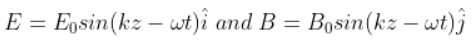Show that,

i) the average energy density of the wave is given by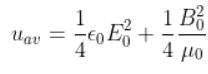ii) the time-averaged intensity of the wave is given by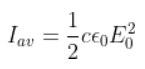i) The energy density due to electric field E is

uE = 1/2 ε0E2

The energy density due to magnetic field B is

uB = 1/2 B20

The average energy density of the wave is given by:ii) We know that c=1/√μ0ε0

The time-averaged intensity of the wave is given asElectromagnetic waves are also called waves which occur in the electromagnetic field containing powerful electromagnetic radiant energy. Electromagnetic radiation is the medium where the waves exist and can traverse through space, moving at the speed of light. Some of the different types of electromagnetic waves are